NEET  >  Test: Rate of a Chemical Reaction

# Test: Rate of a Chemical Reaction

Test Description

## 10 Questions MCQ Test Chemistry Class 12 | Test: Rate of a Chemical Reaction

Test: Rate of a Chemical Reaction for NEET 2022 is part of Chemistry Class 12 preparation. The Test: Rate of a Chemical Reaction questions and answers have been prepared according to the NEET exam syllabus.The Test: Rate of a Chemical Reaction MCQs are made for NEET 2022 Exam. Find important definitions, questions, notes, meanings, examples, exercises, MCQs and online tests for Test: Rate of a Chemical Reaction below.
Solutions of Test: Rate of a Chemical Reaction questions in English are available as part of our Chemistry Class 12 for NEET & Test: Rate of a Chemical Reaction solutions in Hindi for Chemistry Class 12 course. Download more important topics, notes, lectures and mock test series for NEET Exam by signing up for free. Attempt Test: Rate of a Chemical Reaction | 10 questions in 15 minutes | Mock test for NEET preparation | Free important questions MCQ to study Chemistry Class 12 for NEET Exam | Download free PDF with solutions
 1 Crore+ students have signed up on EduRev. Have you?
Test: Rate of a Chemical Reaction - Question 1

### A foreign substance that increase the speed of a chemical reaction is called

Detailed Solution for Test: Rate of a Chemical Reaction - Question 1

Catalyst: Substances which alter  the rate of a chemical reaction and themselves remain chemically and quantitatively unchanged  after the reaction are known as catalysts and the phenomenon is known as catalysis.

Test: Rate of a Chemical Reaction - Question 2

### . What will be the value of instantaneous rate of reaction from the graph?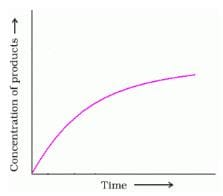Detailed Solution for Test: Rate of a Chemical Reaction - Question 2

If you make a graph of concentration of reactant vs time, the instantaneous reaction rate at a given time is the slope of the tangent line at that point in time. It is also the value of the rate law at a specific concentration (dA/dt is rate = k [A]).

Test: Rate of a Chemical Reaction - Question 3

### The rate of a chemical reaction doubles for every 10°C rise of temperature. If the temperature is raised by 50°C, the rate of the reaction increases by about  [AIEEE 2011]

Detailed Solution for Test: Rate of a Chemical Reaction - Question 3

For 2times=10°c.
for 4times=20°c.
for 6times=30°c.
for 8times=40°c.
and for 10times=50°c.

For every 10°C rise of temperature, the rate is doubled. Thus, the temperature coefficient of the reaction = 2

When temperature is increased by 50°, rate becomes =2(50/10) = 25 times = 32 times

Test: Rate of a Chemical Reaction - Question 4

Chemical substances speeding up rate of chemical reaction is called as

Detailed Solution for Test: Rate of a Chemical Reaction - Question 4

A catalyst is a substance that can be added to a reaction to increase the reaction rate without getting consumed in the process. Catalysts typically speed up a reaction by reducing the activation energy or changing the reaction mechanism. Enzymes are proteins that act as catalysts in biochemical reactions.

Test: Rate of a Chemical Reaction - Question 5

Chemical substances speeding up the rate of chemical reaction is called as

Detailed Solution for Test: Rate of a Chemical Reaction - Question 5

A catalyst is a substance that speeds up the rate of the reaction without being consumed by the reaction itself. When a catalyst is added, the activation energy is lowered because the catalyst provides a new reaction pathway with lower activation energy.

Test: Rate of a Chemical Reaction - Question 6

The rate constant of  zero-order reactions has the unit

Detailed Solution for Test: Rate of a Chemical Reaction - Question 6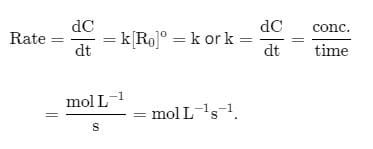Test: Rate of a Chemical Reaction - Question 7

For the reaction A + B → C+ D . The variation of the concentration of the products is given by curve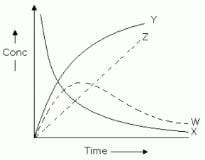Detailed Solution for Test: Rate of a Chemical Reaction - Question 7

For the reaction, A+B⟶C+D, the  variation of the concentration of the products is given by the curve Y.
Initially, the product concentration is 0, then it gradually rises and reaches a maximum value. After that it remains constant.

This behavior is represented by the curve Y.

Test: Rate of a Chemical Reaction - Question 8

The term  -dx/dt  in the rate expression refers to the:

Detailed Solution for Test: Rate of a Chemical Reaction - Question 8

The correct answer is option C
The term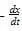in the rate expression refers to the instantaneous rate of the reaction.
It is the ratio of the decrease in the concentration of a reaction to the time taken for this decrease.

Test: Rate of a Chemical Reaction - Question 9

Instantaneous reaction expresses

Detailed Solution for Test: Rate of a Chemical Reaction - Question 9

The correct answer is Option A.

The instantaneous rate is the rate of a reaction at any particular point in time, a period of time that is so short that the concentrations of reactants and products change by a negligible amount.

Test: Rate of a Chemical Reaction - Question 10

Identify A in the given diagram.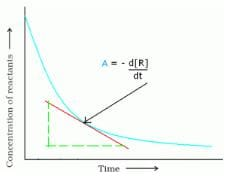Detailed Solution for Test: Rate of a Chemical Reaction - Question 10

The correct answer is Option D
The instantaneous rate is the rate of a reaction at any particular point in time, a period of time that is so short that the concentrations of reactants and products change by a negligible amount. The initial rate is the instantaneous rate of reaction as it starts (as the product just begins to form).

## Chemistry Class 12

157 videos|362 docs|261 tests
 Use Code STAYHOME200 and get INR 200 additional OFF Use Coupon Code
Information about Test: Rate of a Chemical Reaction Page
In this test you can find the Exam questions for Test: Rate of a Chemical Reaction solved & explained in the simplest way possible. Besides giving Questions and answers for Test: Rate of a Chemical Reaction, EduRev gives you an ample number of Online tests for practice

## Chemistry Class 12

157 videos|362 docs|261 tests

### How to Prepare for NEET

Read our guide to prepare for NEET which is created by Toppers & the best Teachers